Next: Some Famous Mathematical Constants Up: Special Numbers and Functions Previous: Why is ?

# Name for f(x)^f(x) = x

Solving for f one finds a ``continued fraction"-like answer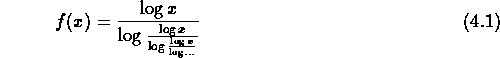This question has been repeated here from time to time over the years, and no one seems to have heard of any published work on it, nor a published name for it.

This function is the inverse of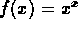. It might be argued that such description is good enough as far as mathematical names go: "the inverse of the function" seems to be clear and succint.

Another possible name is lx(x). This comes from the fact that the inverse of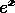is ln(x) thus the inverse of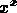could be named lx(x).

It's not an analytic function.

The ``continued fraction" form for its numeric solution is highly unstable in the region of its minimum at 1/e (because the graph is quite flat there yet logarithmic approximation oscillates wildly), although it converges fairly quickly elsewhere. To compute its value near 1/e, use the bisection method which gives good results. Bisection in other regions converges much more slowly than the logarithmic continued fraction form, so a hybrid of the two seems suitable. Note that it's dual valued for the reals (and many valued complex for negative reals).

A similar function is a built-in function in MAPLE called W(x) or Lambert's W function. MAPLE considers a solution in terms of W(x) as a closed form (like the erf function). W is defined as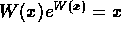.

Notice that f(x) = exp(W(log(x))) is the solution to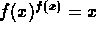An extensive treatise on the known facts of Lambert's W function is available for anonymous ftp at dragon.uwaterloo.ca at /cs-archive/CS-93-03/W.ps.Z.

Alex Lopez-Ortiz
Mon Feb 23 16:26:48 EST 1998